# Team:Tsinghua-A/TeamBITCHINA

Collaborations
with BIT-China

## Help from BIT-China

May 2nd, 2018, we borrowed CEN.PK2-1c modified by Team BIT_China in 2017.Their work in 2017 altered a few steps of the pheromone pathway, which were

• Knocked out Ste2 receptor in order to eliminate the probability of yeasts getting affected by native ligand-pheromone.
• Knocked out Sst2, the GTPase-activating protein for Gpa1p, as a receptor-desensitizing factor in order to eliminate possible up-stream GPCR signal conductive decline due to incessant stimulation. See resource 1 resource 2
• Knocked out Far1, the cyclin-dependent protein serine/threonine kinase inhibitor in order to eliminate side effect to cells’ life circle and formation due to signal intensification in MAPK pathway of the pheromone pathway. More information
• Their modification work provide us with great convenience in our work in 2018.

In August, we borrowed original yeast CEN.PK2-1c from Laboratory Li Chun which Team BIT_China was attached to. The yeast did not undergo modifications below.

• Utilizing α-yeast from the lab of Professor Li Peng as a signal source, we stimulated original yeast imported plasmid of report gene in order to test the function of report plasmid. More information
• We compared the result of ligand combination reaction before and after knocking out the relative gene in yeast, verifying the contributions the modifications made in the elimination of side effect.
• ## How we helped BIT-China

In this year, we make a model based on our research of diagnosis model for Team BIT-China to help them construct the relation between Hydrogen peroxide input and fluorescence intensity.

### Model Background

According to the share of team member in BIT-China, we notice that when the amount of probes are far more than the Hydrogen peroxide. The fluorescence intensity is probably proportion to the concentration of Hydrogen peroxide. Thus, we decided to construct a linear regression model based on the data that Team BIT-China provided to validate the potential linear relationship between fluorescence intensity and Hydrogen peroxide. Also, this model can be use as a standard for Hydrogen peroxide testing.

### Linear regression analysis

#### Definition

$H_2O_2$: The concentration of $H_2O_2$, units in $mM$.

FOD: Florescence intensity / OD

#### Scatter Plot

The Scatter Plot of data are demonstrated below.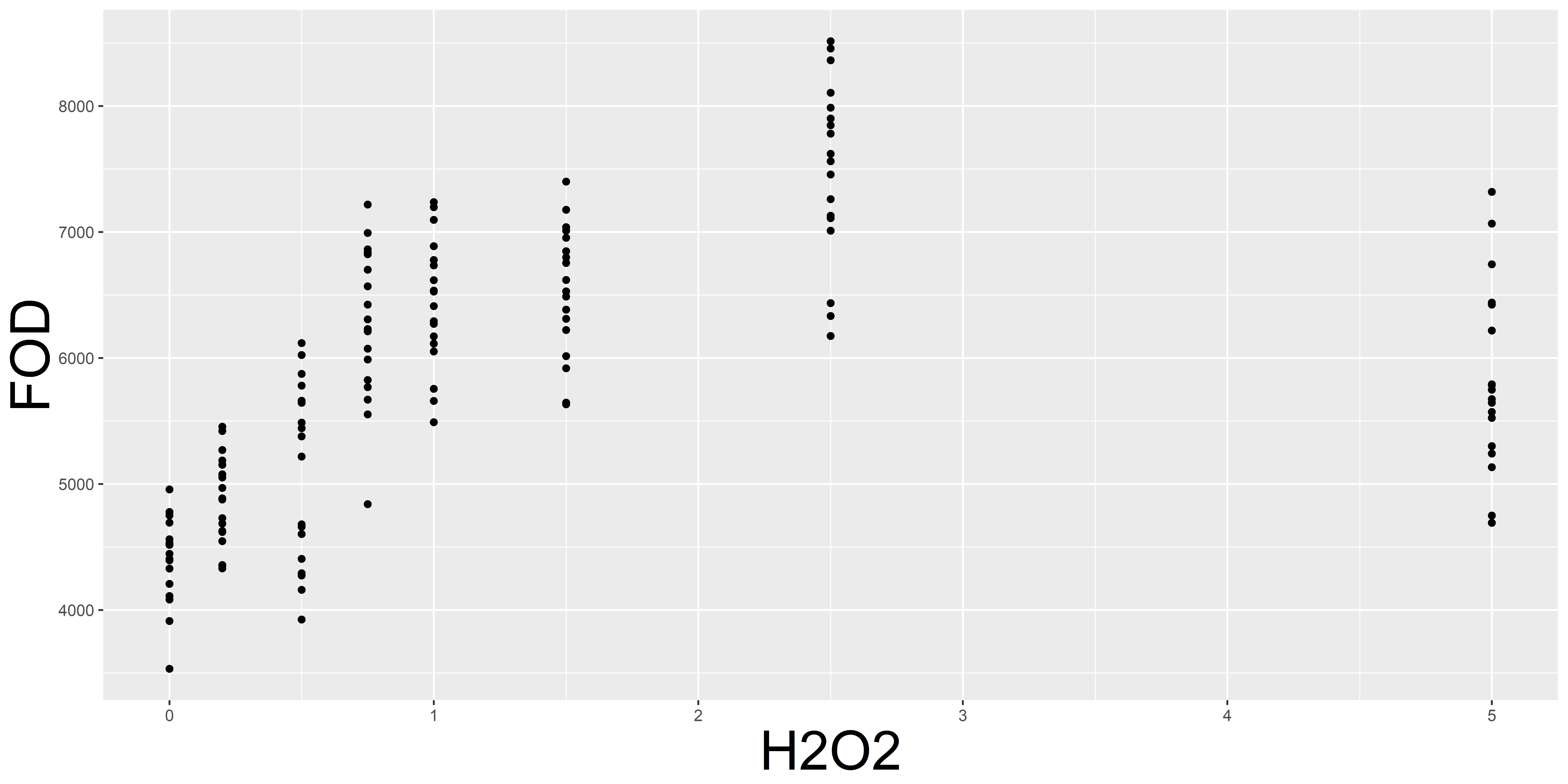From the chart, it is clear that the FOD is in proportion to the $H_2O_2$, when the $H_2O_2$ is between 0-2.5 $mM.$ The data in the points that $H_2O_2$ is 5 are obviously not obey linear model. Thus, we consider to construct a linear model between 0 and 2.5$mM$ of $H_2O_2$. The new Scatter Plot of this part of data are showed below.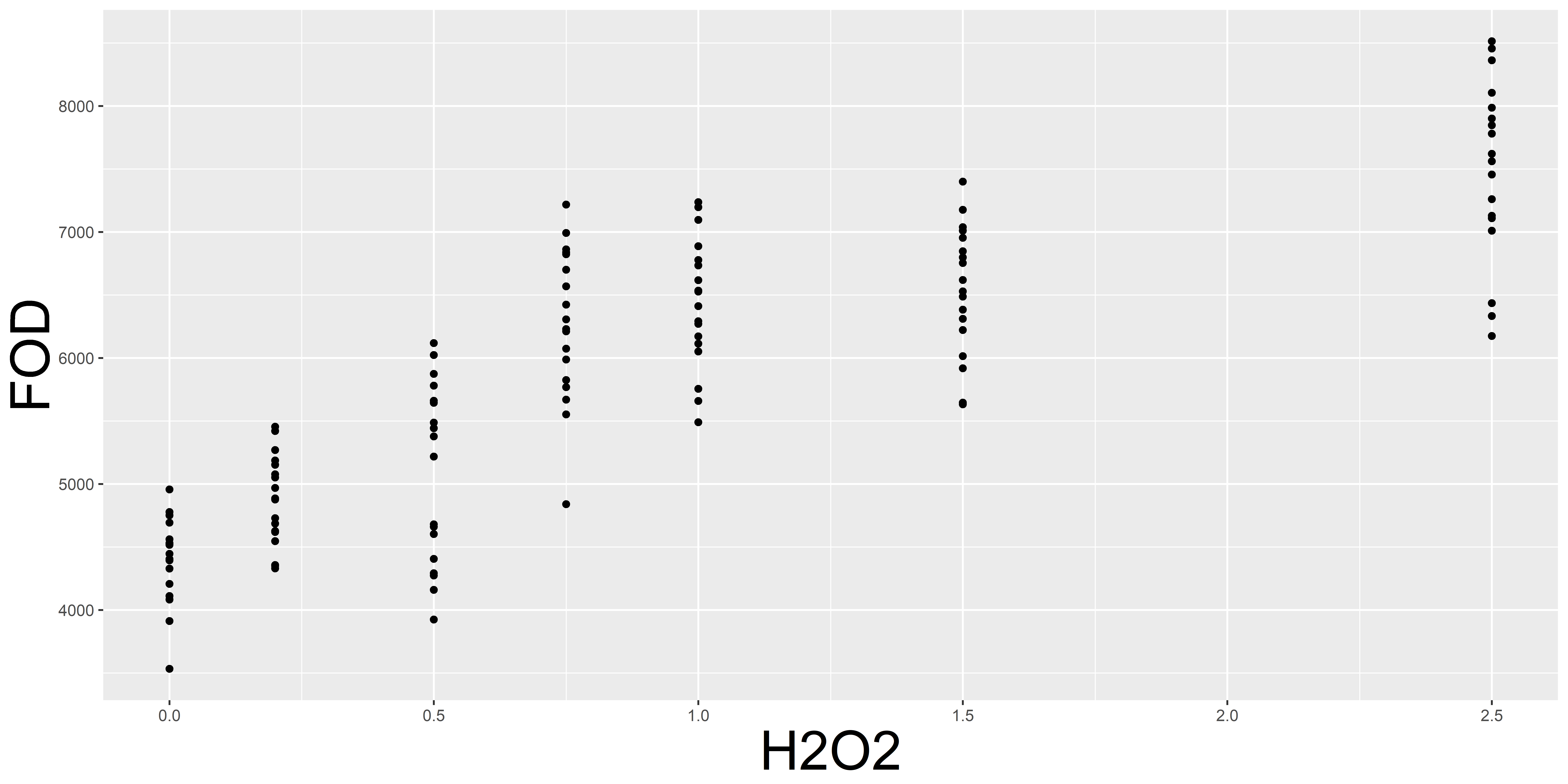The scatter plot witnessed a significant linearity of between FOD and $H_2O_2$, so we tend to use a linear model to predict and explain the results.

### Model Construction

With the criteria of the least square, we construct following linear model

$$FOD_i=β_0+β_1 H_2O_{2i}+ϵ_i ,ϵ_i~N(0,σ^2)$$

The property of the model are demonstrated below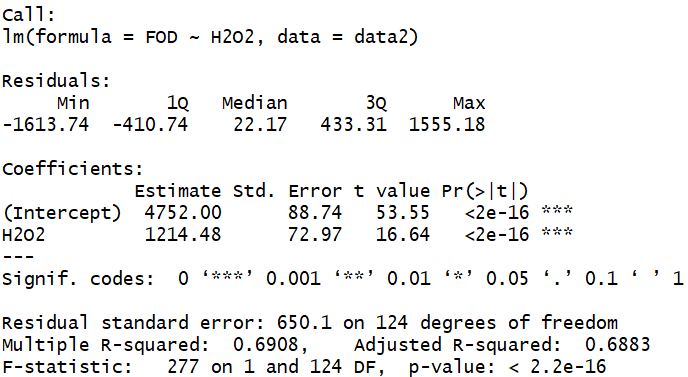It is obvious that the data fits linear model well and the parameters are following

$$FOD_i=4752+1214.48 H_2O_{2i}+ϵ_i,ϵ_i~N(0,425.9)$$

The data along with the fitted values of the model and the confidence interval are pictured below.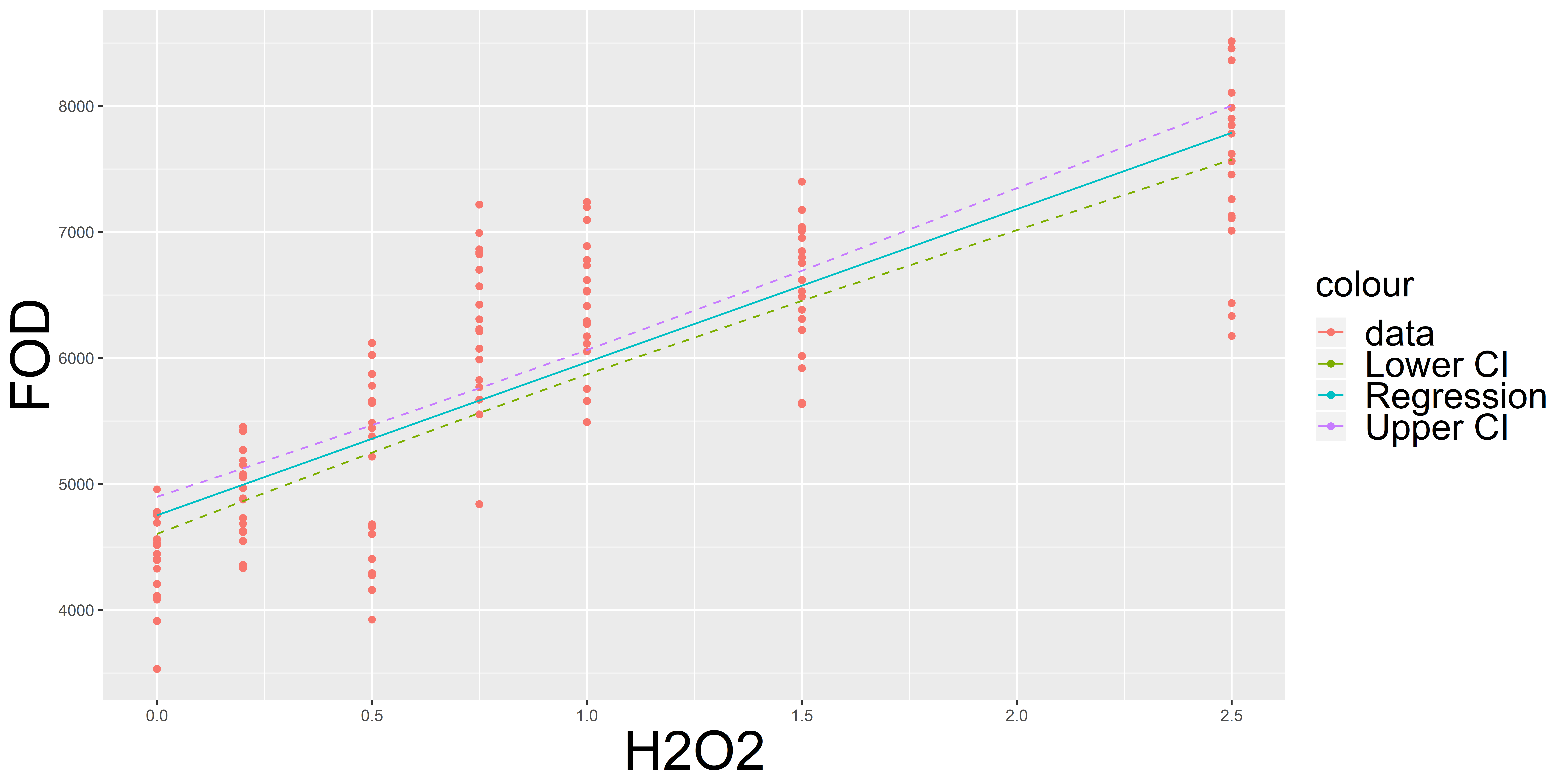### Residuals Analysis

We also run a residuals analysis to ensure this linear model is proper to fit the data. First, we draw histogram and QQ-plot of standard residuals.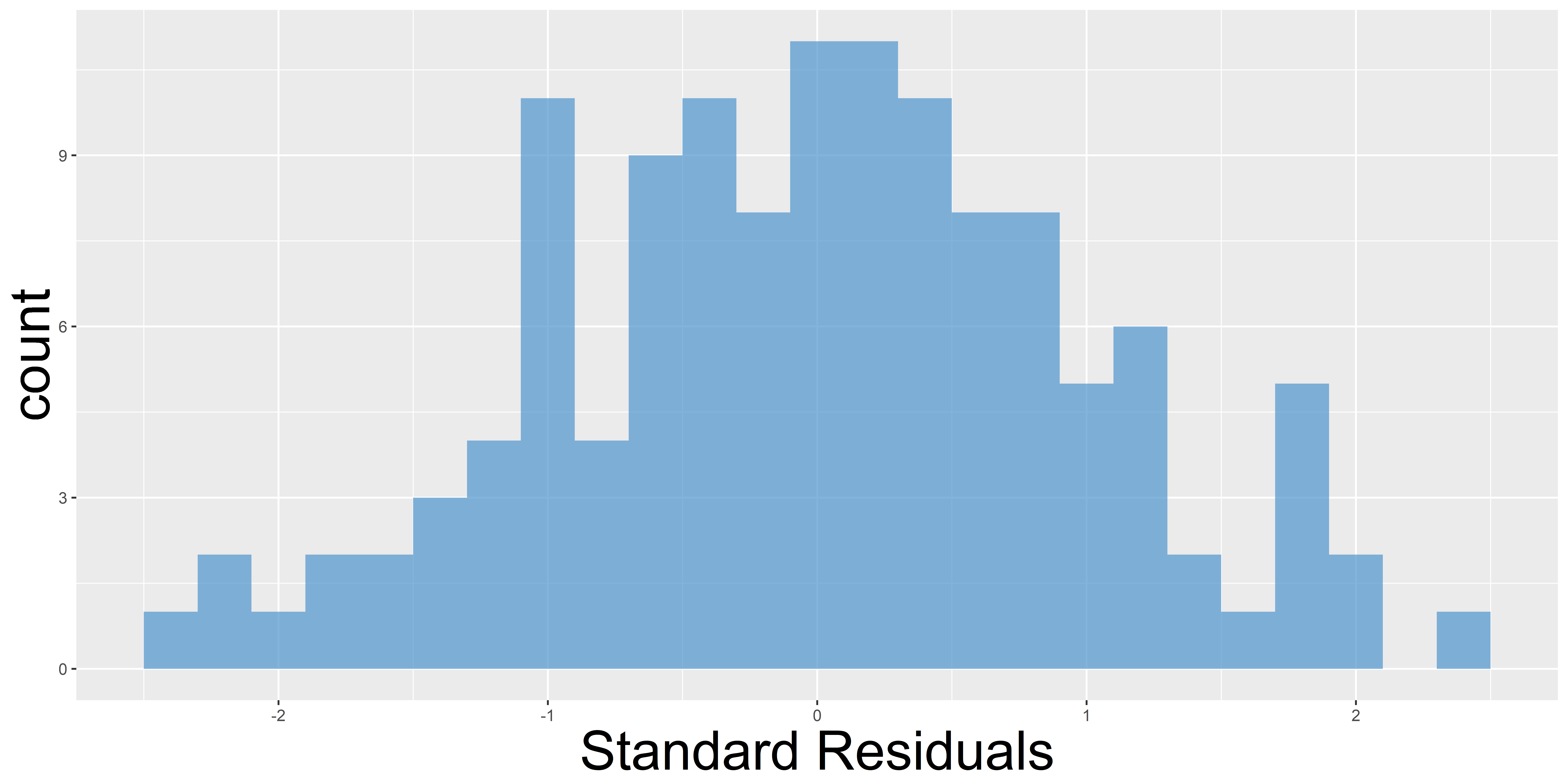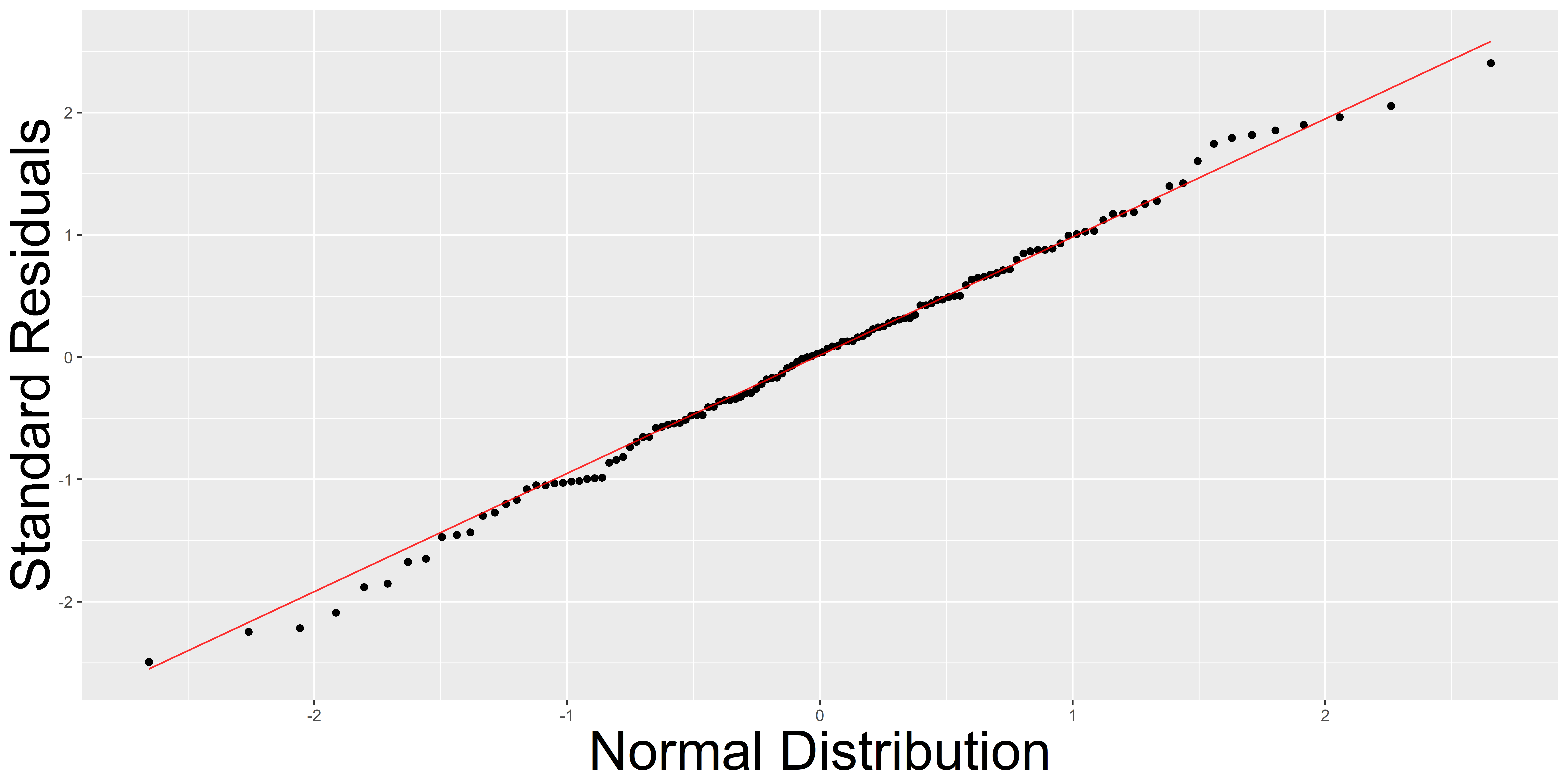From the histogram, the standard residuals equally drop in the two sides of zero, which means that the residuals are possibly independent. Also, the QQ-plot implies the significant normality of residuals.

### Results and inspirations

The linear model we constructed validates the linearity between the concentration of $H_2O_2$ and the fluorescence intensity divided by the OD value, which the $H_2O_2$ is in the interval from 0 to 2.5$mM$. And we also analyze the residuals of the model to ensure it works well. With this model, we can properly predict the output when the concentration of $H_2O_2$ is in certain interval.